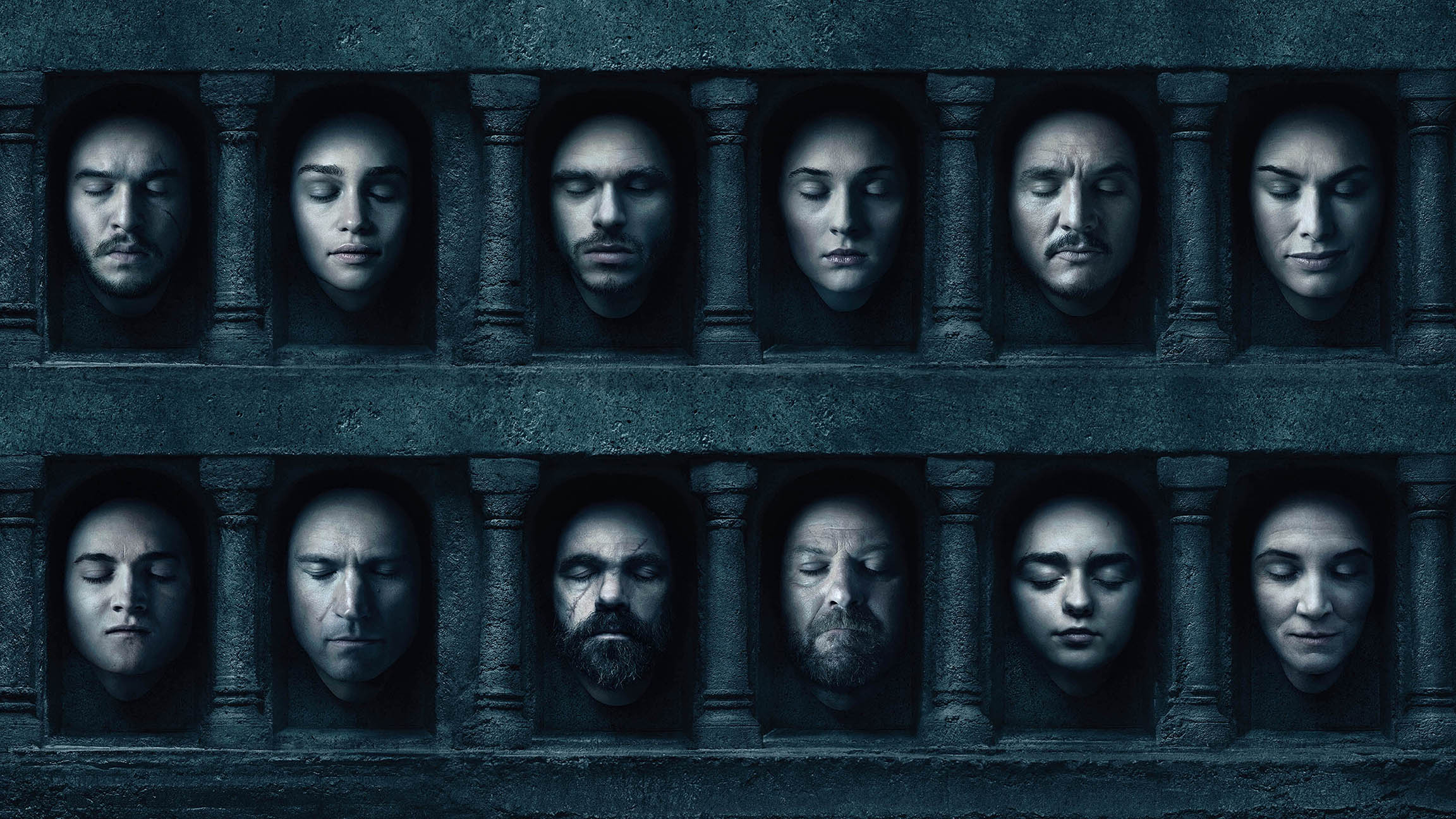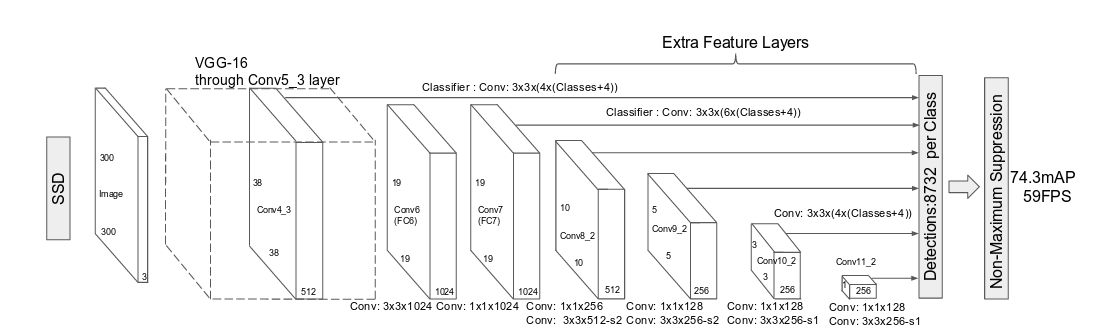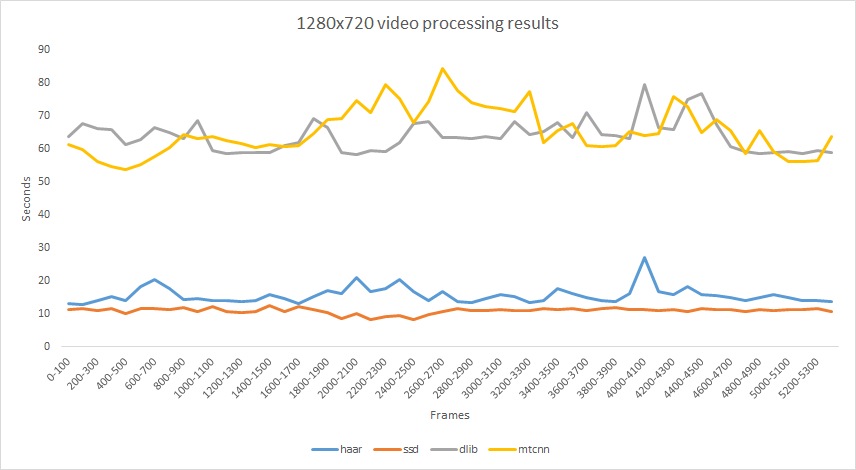# Deep Face Detection with OpenCV in Python

Haven't you subscribe my channel yet?

Follow me on Twitter

Face detection is an early stage of a face recognition pipeline. It plays a pivotal role in pipelines. Herein, deep learning based approach handles it more accurate and faster than traditional methods. In this post, we will use ResNet SSD (Single Shot-Multibox Detector) with OpenCV in Python.Game of Thrones – The Hall of Faces

#### Dependencies

Firstly, the source code of this post is pushed to the GitHub. You can support this study by starring⭐️ the repo.Secondly, we will use external ResNet model and its pre-trained weights provided by OpenCV community. You should download the following files and store in the same directory  you are working.

```#model structure: https://github.com/opencv/opencv/raw/3.4.0/samples/dnn/face_detector/deploy.prototxt
#pre-trained weights: https://github.com/opencv/opencv_3rdparty/raw/dnn_samples_face_detector_20170830/res10_300x300_ssd_iter_140000.caffemodel
```

OpenCV deep neural networks module can load external caffe models.

```detector = cv2.dnn.readNetFromCaffe("deploy.prototxt" , "res10_300x300_ssd_iter_140000.caffemodel")
```

#### Model structure

ResNet SSD model is mainly based on VGG.ResNet SSD

#### Loading the image

SSD model expects you to feed (300, 300, 3) sized inputs. We will resize the input images to 300×300 but this is a low resolution and not to lose resolution I also store the base image in a different variable.

```image = cv2.imread("image.jpg")
base_img = image.copy()
original_size = base_img.shape
target_size = (300, 300)
image = cv2.resize(image, target_size)
aspect_ratio_x = (original_size / target_size)
aspect_ratio_y = (original_size / target_size)
```

That’s going to be the image we will process.Arya Stark and Jon Snow in Game of Thrones

We’ve already resized the base image to 300×300 pixels but OpenCV actually expects you to feed (1, 3, 300, 300) sized inputs exactly. The easier way is to use blobFromImage function. If you want to code this logic, then you can alternatively roll the 3rd dimension to 1st, then add a dummy dimension to the left by calling expand dimensions function.

```#detector expects (1, 3, 300, 300) shaped input
imageBlob = cv2.dnn.blobFromImage(image = image)
#imageBlob = np.expand_dims(np.rollaxis(image, 2, 0), axis = 0)
```

#### Feed forward

We can feed the processed image to the caffe model now. This is a basic feed forward step in neural networks.

```detector.setInput(imageBlob)
detections = detector.forward()
```

#### Focusing on strong face candidates

The output of the neural networks is (200, 7) sized matrix. Here, rows refer to candidate faces whereas columns state some features. We will filter these candidate faces based on its features.

```column_labels = ["img_id", "is_face", "confidence", "left", "top", "right", "bottom"]
detections_df = pd.DataFrame(detections, columns = column_labels)
```Detections

Feature is_face will be 0 for background and it will be 1 for face instances. That’s why, we will ignore the zero values. We will also ignore the instances having a confidence value less than a threshold value (e.g. 90%).

```#0: background, 1: face
detections_df = detections_df[detections_df['is_face'] == 1]
detections_df = detections_df[detections_df['confidence']&amp;amp;amp;amp;lt;=0.90]
```

Coordinate values – left, right, top, bottom – are in [0, 1]. Remember that we’ve resized the input image to (300, 300). We should multiple those coordinate values to 300 to find the exact location in resized image.

```detections_df['left'] = (detections_df['left'] * 300).astype(int)
detections_df['bottom'] = (detections_df['bottom'] * 300).astype(int)
detections_df['right'] = (detections_df['right'] * 300).astype(int)
detections_df['top'] = (detections_df['top'] * 300).astype(int)
```

Applying those filters eliminates most of instances and the following two instances appear in the pandas data frame.Found faces

#### Plotting

We can extract the detected face area from the base image.

```for i, instance in detections_df.iterrows():
confidence_score = str(round(100*instance["confidence"], 2))+" %"
left = instance["left"]; right = instance["right"]
bottom = instance["bottom"]; top = instance["top"]
detected_face = base_img[int(top*aspect_ratio_y):int(bottom*aspect_ratio_y) ,
int(left*aspect_ratio_x):int(right*aspect_ratio_x)]
print("Id ",i,". Confidence: ", confidence_score)
plt.imshow(detected_face[:,:,::-1])
plt.show()
```Detected faces

We can focus on the detected faces in the base image as well.

```cv2.putText(base_img, confidence_score, (int(left*aspect_ratio_x), int(top*aspect_ratio_y-10)), cv2.FONT_HERSHEY_SIMPLEX, 0.5, (255, 255, 255), 1)
cv2.rectangle(base_img, (int(left*aspect_ratio_x), int(top*aspect_ratio_y)), (int(right*aspect_ratio_x), int(bottom*aspect_ratio_y)), (255, 255, 255), 1) #draw rectangle to main image
```Deep Face Detection

#### Face detectors

There are several face detection solutions. Firstly, OpenCV offers Haar Cascade and Single Shot Multibox Detector (SSD). Besides, Dlib offers Histogram of Oriented Gradients (HOG) and Max-Margin Object Detection (MMOD). Finally, Multi-task Cascaded Convolutional Networks (MTCNN) is a popular solution nowadays. Herein, Haar Cascade and HoG are legacy methods whereas SSD, MMOD and MTCNN are deep learning based modern methods.

Face detection score is more accurate in SSD and MTCNN. I cannot test MMOD because it requires a very powerful hardware. The following video show the comparison of those techniques. False positive rates are high in Haar Cascade and HoG. They can detect non-facial objects such as tie or badge as faces. SSD and MTCNN give more robust results.

Here, you can find the source code of this real time study. It wraps all of those state-of-the-art face detection implementations.

SSD is the fastest one. I’ve tested those models on a 720p video on my i7 laptop. Averagely, SSD can process 9.20 frames per second whereas haar cascade can handle 6.50 fps, dlib HoG can run 1.57 fps and mtcnn can do 1.54 fps. It seems that SSD is the most robust and fastest one among others.Face detector performances

Deepface already wraps those face detectors. detectFace function applies detection and alignment respectively.

```from deepface import DeepFace backends = ['opencv', 'ssd', 'dlib', 'mtcnn']
for backend in backends:
detected_aligned_face = DeepFace.detectFace(img_path = "img.jpg" , detector_backend = backend)
```

Here, you can watch how to use different face detectors in Python.

Alternatively, you can apply detection and alignment manually.

```img = functions.load_image("img.jpg")
backends = ['opencv', 'ssd', 'dlib', 'mtcnn']
backend = backends
detected_face = functions.detect_face(img = img, detector_backend = backend)
aligned_face = functions.align_face(img = img, detector_backend = backend)
processed_img = functions.detect_face(img = aligned_face, detector_backend = backend)
plt.imshow(processed_img)
```

Herein, retinaface is the cutting-edge technology for face detection. It can even detect faces in the crowd. Besides, it finds some facial landmarks including eye coordinates. In this way, its alignment score is high as well.

#### Face recognition pipeline

Face detection is the 1st stage of a modern face recognition pipeline. The following video guides you to build a pipeline end to end.

#### Conclusion

So, we’ve mentioned how to apply face detection with deep neural networks approach within OpenCV in Python. This approach comes with a more accurate and faster results than traditional haar cascade method.

I pushed the source code of this study to GitHub. You can support this study by starring⭐️ the repo.

Like this blog? Support on PatreonThis site uses Akismet to reduce spam. Learn how your comment data is processed.